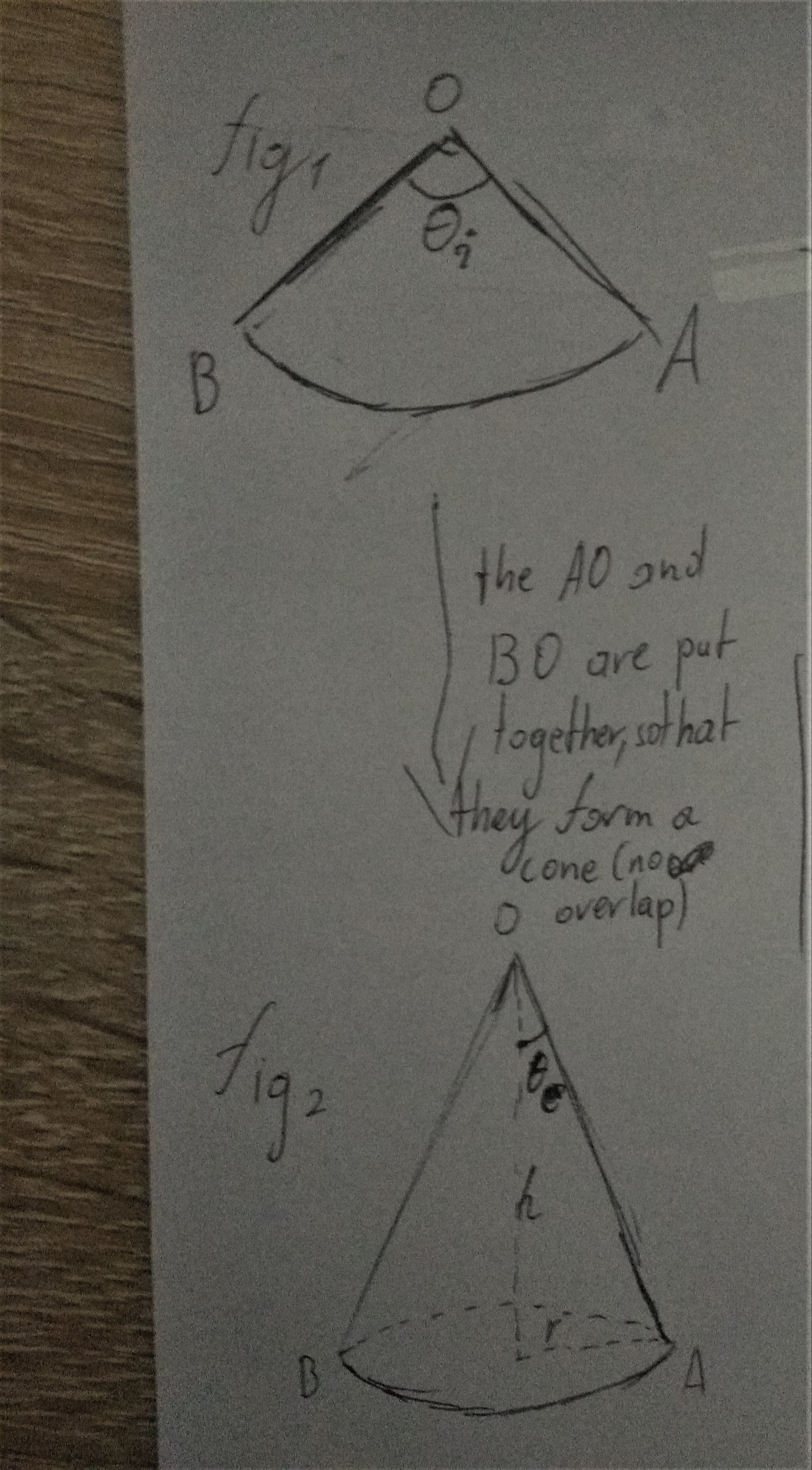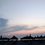# Ever wondered what is the relation of these angles?

This formula derivation was inspired by a math exercise I did in class (Which is weird cause the exercises in Math are almost all elementary).

You need to know basic trigonometry for this one.

The formula does:

$\boxed{\text{Input:} \theta_{i}}$

$\boxed{\text{Output:} \theta_{e}}$

This photo initializes the variables used for the following proof:Note that $\theta_{i}$ can obtain such values that $[0 < \theta_{i} < 360 ]$ . $360$ is just a full circle and beyond it is overlap.

To find $\theta_{e}$ we need to relate it between the $h$ and $OA$:

$\cos (\theta_{e}) = \frac{h}{OA}$

By the $\text{Pythagorean theorem}$:

$h^{2} + r^{2} = OA^{2}$

$h= \sqrt{OA^{2} - r^{2}}$

Replace $h$ in the $\cos (\theta_{e}) = \frac{h}{OA}$ we get:

$\cos (\theta_{e}) = \frac{\sqrt{OA^{2} - r^{2}}}{OA}$

We can find $r$ by making a relation with the surface of the 2 objects which does not change during transformation from $\text{fig.1}$ to $\text{fig.2}$; call this $S_{side}$. $S_{side}$ can be found in 2 ways:

• $S_{side} = \pi \times (OA)^{2} \times \frac{ \theta_{i} }{360}$ , by $\text{fig.1}$

• $S_{side} = \pi \times (OA) \times r$ , by $\text{fig.2}$

Let's equal these 2 expressions:

$\pi \times (OA) \times r = \pi \times (OA)^{2} \times \frac{ \theta_{i} }{360}$ We can simplify this to:

$r = (OA) \times \frac{ \theta_{i} }{360}$

Replace $r$ in the $\cos (\theta_{e}) = \frac{\sqrt{OA^{2} - r^{2}}}{OA}$ we get:

$\cos (\theta_{e}) = \frac{\sqrt{OA^{2} - ((OA) \times \frac{ \theta_{i} }{360})^{2}}}{OA}$

Let's simplify this expression. Using the property $a^{2} - b^{2} = (a+b)(a-b)$ in $\sqrt{OA^{2} - ((OA) \times \frac{ \theta_{i} }{360})^{2}}$:

$\cos (\theta _{ e })=\frac { \sqrt { ([OA]+[OA]\times \frac { \theta _{ i } }{ 360 } )\times ([OA]-[OA]\times \frac { \theta _{ i } }{ 360 } ) } }{ OA }$

I did it in square brackets "$[OA]$" to not confuse too much the reader.

Factorise $([OA]+[OA]\times \frac { \theta _{ i } }{ 360 } )$ and in $([OA]-[OA]\times \frac { \theta _{ i } }{ 360 } )$ a $[OA]$:

$\cos (\theta _{ e })=\frac { \sqrt { { [OA]^{ 2 }(1+\frac { \theta _{ i } }{ 360 } )(1-\frac { \theta _{ i } }{ 360 } ) } } }{ OA }$

The $[OA]$ -s cancel out, so we are left with the final formula (Yes, $\theta _{ e }$ is independent from $[OA]$) :

## $\boxed {\cos (\theta _{ e })=\sqrt { { (1+\frac { \theta _{ i } }{ 360 } )(1-\frac { \theta _{ i } }{ 360 } ) } } }$

Quite an elegance!Note by Nikolas Кraj
7 months ago

This discussion board is a place to discuss our Daily Challenges and the math and science related to those challenges. Explanations are more than just a solution — they should explain the steps and thinking strategies that you used to obtain the solution. Comments should further the discussion of math and science.

When posting on Brilliant:

• Use the emojis to react to an explanation, whether you're congratulating a job well done , or just really confused .
• Ask specific questions about the challenge or the steps in somebody's explanation. Well-posed questions can add a lot to the discussion, but posting "I don't understand!" doesn't help anyone.
• Try to contribute something new to the discussion, whether it is an extension, generalization or other idea related to the challenge.
• Stay on topic — we're all here to learn more about math and science, not to hear about your favorite get-rich-quick scheme or current world events.

MarkdownAppears as
*italics* or _italics_ italics
**bold** or __bold__ bold
- bulleted- list
• bulleted
• list
1. numbered2. list
1. numbered
2. list
Note: you must add a full line of space before and after lists for them to show up correctly
paragraph 1paragraph 2

paragraph 1

paragraph 2

[example link](https://brilliant.org)example link
> This is a quote
This is a quote
    # I indented these lines
# 4 spaces, and now they show
# up as a code block.

print "hello world"
# I indented these lines
# 4 spaces, and now they show
# up as a code block.

print "hello world"
MathAppears as
Remember to wrap math in $$ ... $$ or $ ... $ to ensure proper formatting.
2 \times 3 $2 \times 3$
2^{34} $2^{34}$
a_{i-1} $a_{i-1}$
\frac{2}{3} $\frac{2}{3}$
\sqrt{2} $\sqrt{2}$
\sum_{i=1}^3 $\sum_{i=1}^3$
\sin \theta $\sin \theta$
\boxed{123} $\boxed{123}$

## Comments

Sort by:

Top Newest

Please report here for any mistake i have done (in reply here). Thanks

- 7 months ago

Log in to reply

×

Problem Loading...

Note Loading...

Set Loading...